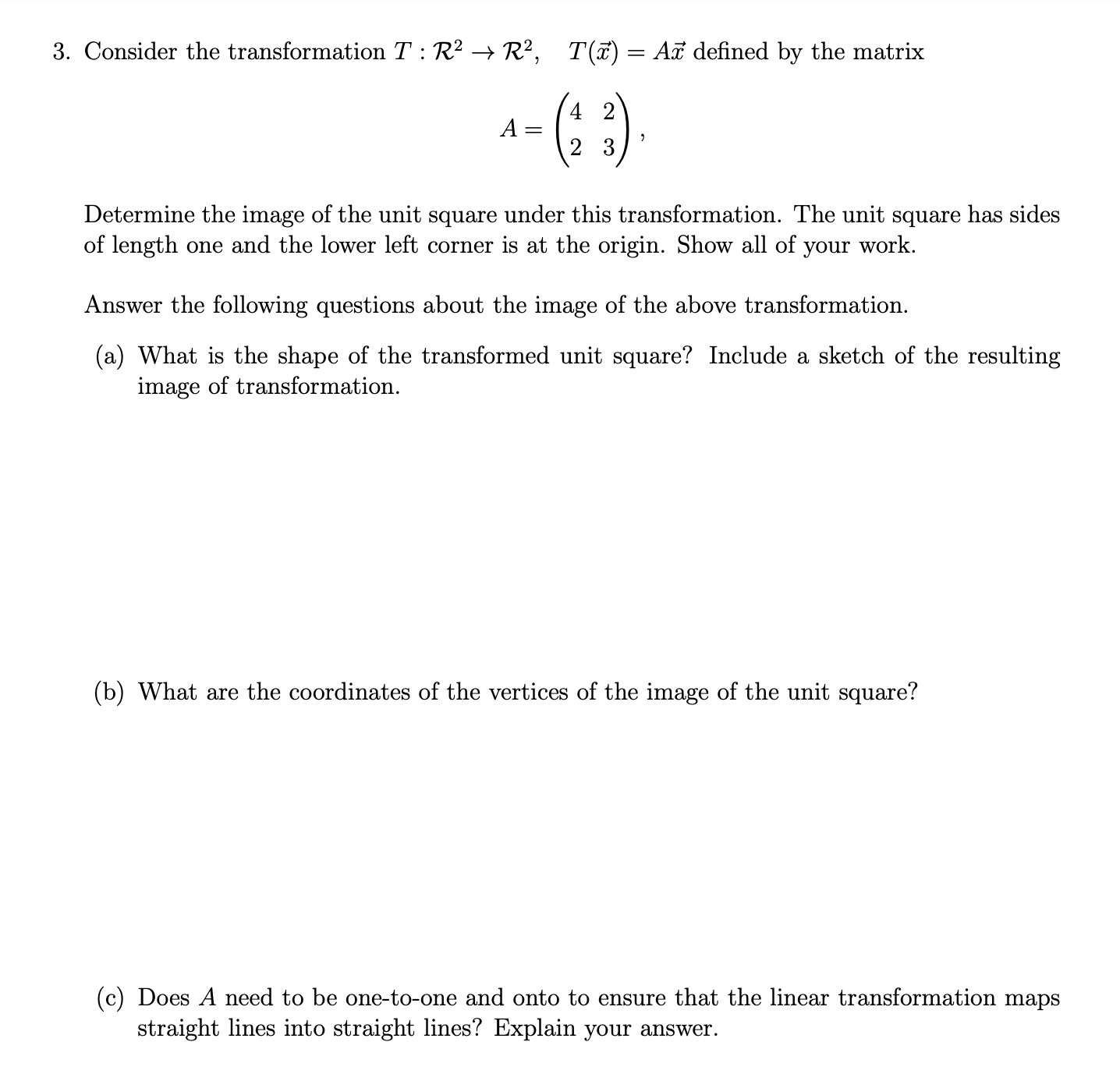# (Solved): 3. Consider the transformation T:R2R2,T(x)=Ax defined by the matrix A=(4223), Determine th ...3. Consider the transformation defined by the matrix Determine the image of the unit square under this transformation. The unit square has sides of length one and the lower left corner is at the origin. Show all of your work. Answer the following questions about the image of the above transformation. (a) What is the shape of the transformed unit square? Include a sketch of the resulting image of transformation. (b) What are the coordinates of the vertices of the image of the unit square? (c) Does need to be one-to-one and onto to ensure that the linear transformation maps straight lines into straight lines? Explain your answer.

We have an Answer from Expert